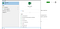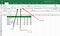# The Basics Of Excel

Excel is a wonderful tool designed by Microsoft to work with Tabular data. The power of excel is great and it is being used by many industries. It doesn’t matter from which domain you belong Excel works really well like the magic stick of Harry Porter.

Let's start with an example.

Example 1: A accountant uses Excel to manage the financial data in his/her domain.

Example 2: A data scientist or Computer engineer uses excel to preprocess data, Clean the data, Automation stuff, Creating SQL-based Queries and the list of uses is unending.

Let's see how to open an excel in windows and how it looks like.

• Press the Window key, A Search tab will open like this.How to open excel
• Type Excel in the search bar and click on excel which is installed on your computer.

A new window will be open like this. This contains the sheet and the data in tabular format. We can click on the cells.

Each cell having its address, Row defines in the number (0–9)and Column defines the Characters (A-Z). By the combination of two figures become one address of the cells. For Example, as per the above diagram, the selected cell has address A1 which we also can see in the address bar.

We can click on the cells and enter the data in them using the keyboard. As an example sample data entered of some students.

To Add the Background color, Font color, Border use the below mentioned instruction.Border, Background color settings in excel

How to Apply functions in Excel?

Functions are very important in excel. It's used for the manipulation and calculation of the data like sum, multiply, Division, etc. There are many types of functions are available in excel.

Generally, the functions are divided into categories:

1. Built-in functions: These are those functions which are already available in the excel. We can direcly use in our projects. for example sum, min, max, multiply etc.
2. User Defined functions: These are those functions which user can define as per the custom requirement.

Lets see how to use the built in function : In this example we are going to apply the sum function.

• Click on the cell where you want to apply the function and enter the formula in the formula tab.
• To apply a function there should be 3 things in the mind.
1. Function starts with = symbol
2. After = enter the function name
3. Select the range up to where you want to apply the function

=FunctionName(Range)

=sum(StartAddress:EndAddress)

As per the above example, we have applied the formula. =sum(D2:D6), whereas the D2:D6 is the start address and the end address. it will sum the d2 to d6 and the result will get stored in the selected cells.

in this way, we can almost every function. min(), max(), average() and the list of the functions are unending so choose the function as per the requirement.

• SUM() : Sum function is used to add 2 or more numbers/values.
• Average(): Average Function is used to find the average of 2 or more values/numbers.
• Max(), Min(): Max, Min Function is used to finding maximum or minimum between 2 or more values.

Click here : To get the list of commonly used functions in excel and have some experiments as per the usage.

Hope you enjoyed this small blog. The credit of blogs to Kamaljeet Singh.

Click here to Download the excel used in this session.

:) Happy coding :D

We let make your business smarter and broader through the power of the internet. Researcher | Web developer | Internet of things | AI | www.wakeupcoders.com

## More from Wakeupcoders

We let make your business smarter and broader through the power of the internet. Researcher | Web developer | Internet of things | AI | www.wakeupcoders.com